# Covered Call Strategy Using Machine LearningA covered call is used by an investor to make some small profit while holding the stock. Mostly the reason why a trader would want to create a covered call is because the trader is bullish on the underlying stock and wants to hold for long-term, but the stock doesn't pay any dividend.The stock is expected to go up over a period of next 6 months, and in the meantime, you would want to use this stock as collateral and sell some call and pocket the premium. But there is a risk to the strategy, that is if the stock goes up then your stock would get called away at expiry. So, instead of waiting for the option to expire, you can buy it back for a lesser premium.

There are many ways to use machine learning for trading. In this blog, I will try to show you how you could benefit by using a simple decision tree algorithm to predict a short-term move in the option premium price and pocket the difference while holding the stock.

For more details on different option trading strategies please visit https://quantra.quantinsti.com/

Let me show you an example, using the Nifty futures. Nifty50 is an Indian Index comprising of 50 stocks from different sectors.

To execute the above-discussed strategy, we assume that we are holding the futures contract and then we try to write a call option on the same underlying. To do this, we train a machine learning algorithm on the past data consisting of various greeks, such as IV, delta, gamma, vega, and theta of the option as the input. And the dependent variable or the prediction would be made on the next day’s return. We write the call whenever the algorithm generates a sell signal. To begin with, let us import the necessary libraries.

### Importing the Libraries

```import pandas as pd import mibian as mb from sklearn.metrics import accuracy_score from sklearn.tree import DecisionTreeClassifier import matplotlib.pyplot as plt import numpy as np```

Mibian is the library that I used to calculate the option greeks using the Black Scholes Model.

First, let us import the data. I have two datasets, one with the continuous data of the Futures Contract and another with the continuous data of the 9000 strike call option. Hereby continuous I mean that is across various expiries.

### Importing the Data

```futures_data= pd.read_csv("Future_data.csv") options_data= pd.read_csv("Option_data.csv")```

The data in the csv file used in this blog is downloaded from the NSE website.
Let us print the data sets to visualize them.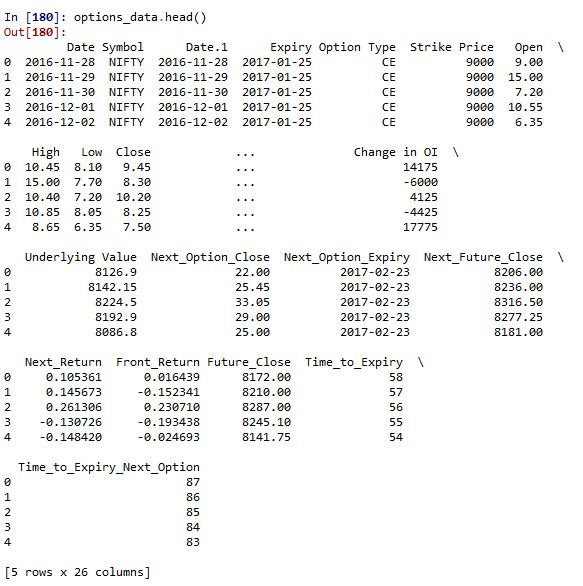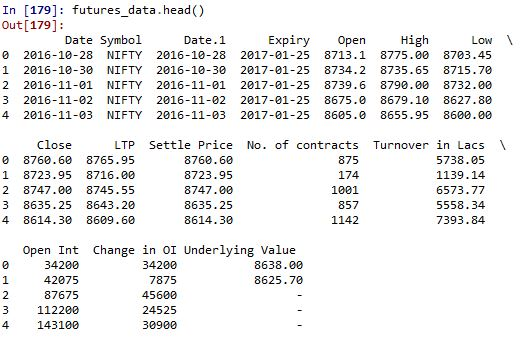As you can see below, we have data starting from 26th of October for the futures data and 26th of November for the Options data. Let us analyse the length of the datasets we have.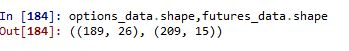### Merging the Data

So, we need to take only that data from the two files which are used for prediction and for only those dates which are present in both the datasets.

```futures_data['Date'] = pd.to_datetime(futures_data['Date']) options_data['Date'] = pd.to_datetime(options_data['Date'])```

```futures_data=futures_data.loc[futures_data.Date.isin(options_data.Date)] options_data=options_data[options_data.Date.isin(futures_data.Date)]```

Now take only those features that we need to calculate the greeks.

```futures_data=futures_data[['Date','LTP']] options_data=options_data[['Date','LTP','Time_to_Expiry']]```

After this, we will merge the two data frames over the Date column. But to do that we need to change the names of the LTP columns, which is same in both the data frames.

```futures_data.rename(index=str, columns={"LTP": "Fut_LTP"}, inplace=True) options_data.rename(index=str, columns={"LTP": "Opt_LTP"}, inplace=True)```

`Nifty=pd.merge(futures_data,options_data,how='inner')`

### Calculating the Greeks

Now, let us save the column names in a list, as we will be dropping these columns once the greeks are calculated and use them to make predictions.

`col=list(Nifty.columns.values)`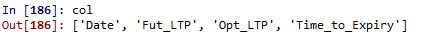Next, we use the mibian library to calculate the greeks, and we will do this without using the for-loop.

`Nifty['IV']=Nifty.apply(lambda a: mb.BS([a['Fut_LTP'],9000,0,a['Time_to_Expiry']],callPrice=a['Opt_LTP']).impliedVolatility, axis=1)`

`Nifty['Delta']=Nifty.apply(lambda a: mb.BS([a['Fut_LTP'],9000,0,a['Time_to_Expiry']],volatility=a['IV']).callDelta, axis=1)`

`Nifty['Vega']=Nifty.apply(lambda a: mb.BS([a['Fut_LTP'],9000,0,a['Time_to_Expiry']],volatility=a['IV']).vega, axis=1)`

`Nifty['Gamma']=Nifty.apply(lambda a: mb.BS([a['Fut_LTP'],9000,0,a['Time_to_Expiry']],volatility=a['IV']).gamma, axis=1)`

`Nifty['Theta']=Nifty.apply(lambda a: mb.BS([a['Fut_LTP'],9000,0,a['Time_to_Expiry']],volatility=a['IV']).callTheta, axis=1)`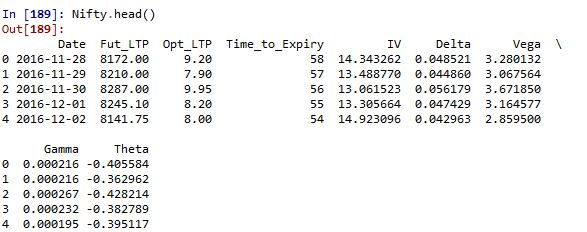### Generating the Signal

After this, we will create a signal column, this is the dependent variable that our algorithm will learn to predict. This signal will generate a sell signal every time the market closes lower the next day. This signal will not be a part of the input data and hence will be added to our col list.

```Nifty['Signal']=0 Nifty['Signal'].loc[Nifty.Opt_LTP.shift(-1)<Nifty.Opt_LTP]=-1```

```col.append('Signal') Nifty=Nifty.dropna()```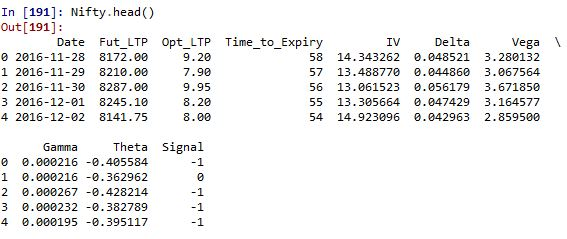After this, we will we create the independent and dependent variable. In our case, the Greeks are the parameters which the algorithm will learn and Signal is the variable that the algorithm will predict. Let us denote these two datasets by X and y.

```X=Nifty.drop(col,axis=1) y=Nifty.Signal```

### Splitting the Data

Next, we will use the first 95% of the data as the train data and the last 5% for prediction. So, we will use the first 179 days data for training the algorithm and last 10 days ( 2 weeks) of trading data to predict its performance.

`split=int(0.95*(len(X)))`

`X_train, X_test, y_train, y_test = X[:split],X[split:],y[:split],y[split:]`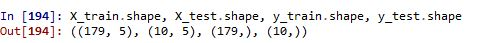### Fitting the Decision Tree

Next, we instantiate a sample decision tree and fit the train data to make predictions on the test data. We will evaluate the performance of the strategy by calculating the returns (in terms of call premium) of the strategy and then adding every day’s return for the data in the test dataset.

```cls=DecisionTreeClassifier() cls.fit(X_train,y_train)```

```Nifty['Pred_Signal']=cls.predict(X) Nifty['Return']=Nifty.Opt_LTP.shift(-1)-Nifty.Opt_LTP Nifty['Str_Return']=Nifty.Pred_Signal*Nifty.Return```

`print(accuracy_score(y_test,cls.predict(X_test)))`

```plt.plot(Nifty.Str_Return.iloc[X_test.index].values.cumsum()) print(np.nansum(Nifty.Str_Return.iloc[X_test.index].values)) plt.show()```

We will also print the accuracy and profit of the strategy.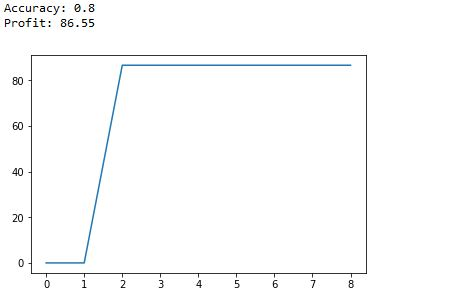Depending on the random state of the algorithm, the profit results might vary, but the accuracy would be close to the value above.

### Next Step

Learn about the Candlestick trading strategy. We have explained a momentum strategy where you observe price on the previous ‘n’ candlesticks and make your bets accordingly.